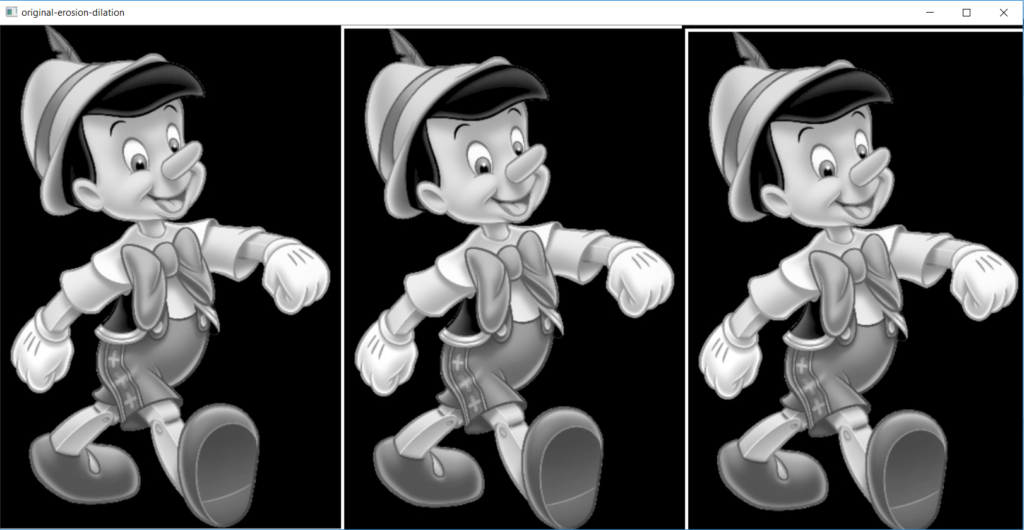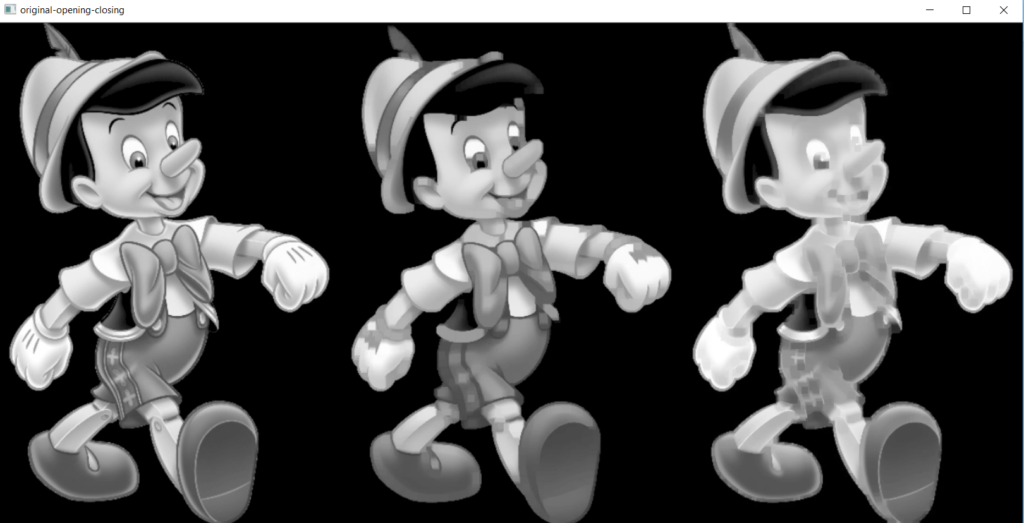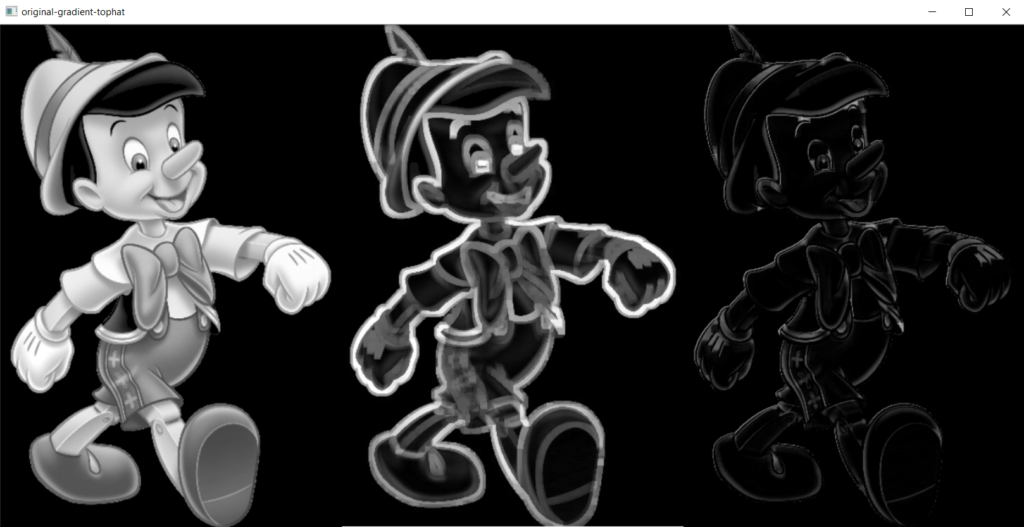# Morphological Operation

Morphology is a branch in which we study the shape and size of an object or in other words, we may say we find the structure of the object. We use the concept of structuring element in this. So what is Structuring Element?.

Structuring Elements is a mask or window which is placed on the original image to find the desired output.

There are two main characteristics of structuring element.

Shape: It may be circular, triangle, or rectangle.

Size: Varies from 3×3 to 2×2 etc..

Morphological operation are of following types:

1-Dialation

2-Erosion

3-Opening

4-Closing

### Dilation

This operation consists of convoluting an image A with some kernel (B), which can have any shape or size, usually a square or circle. Dilation generally change the pixel value

To perform dilation there are two methods: 1)-Substitution 2)-Vector addition.

### Erosion

In this, all the pixels near boundary will be discarded depending upon the size of the kernel. So the thickness or size of the foreground object decreases or simply white region decreases in the image. Characterstic of erosion are:

1)Remove the noise. 2)-Decreases the brightness. 3)-Keep the image same.

Code:

```import cv2
import numpy as np

kernel = np.zeros((2,2),np.uint8)

erosion = cv2.erode(img,kernel,iterations = 1)
dilation = cv2.dilate(img,kernel,iterations = 1)
numpy_horizontal = np.hstack((img,erosion, dilation))

cv2.imshow("original-erosion-dilation", numpy_horizontal)
cv2.waitKey(0)
cv2.destroyAllWindows()```### Opening

The opening is just another name of erosion followed by dilation. It means first cut the corner and then expand.

### Closing

Closing is reverse of Opening, Dilation followed by Erosion. It means first expand then cut the border.

Code:

```import cv2
import numpy as np

kernel = np.ones((8,8),np.uint8)

opening = cv2.morphologyEx(img, cv2.MORPH_OPEN, kernel)
closing = cv2.morphologyEx(img, cv2.MORPH_CLOSE, kernel)
numpy_horizontal = np.hstack((img,opening,closing))

cv2.imshow("original-opening-closing", numpy_horizontal)
cv2.waitKey(0)
cv2.destroyAllWindows()```

output:There are so many other morphological operations like:

It is the difference between dilation and erosion of an image.

### Top Hat

It is the difference between the input image and Opening of the image.

Code:

```import cv2
import numpy as np

kernel = np.ones((8,8),np.uint8)

tophat = cv2.morphologyEx(img, cv2.MORPH_TOPHAT, kernel)

cv2.waitKey(0)
cv2.destroyAllWindows()```

Output:Refer:

https://docs.opencv.org/3.0-beta/doc/py_tutorials/py_imgproc/py_morphological_ops/py_morphological_ops.html

https://homepages.inf.ed.ac.uk/rbf/HIPR2/erode.htm

https://homepages.inf.ed.ac.uk/rbf/HIPR2/dilate.htm